# AP Statistics : How to do the linear transformation of a random variable

## Example Questions

Explanation:

Explanation:

### Example Question #2 : How To Do The Linear Transformation Of A Random Variable

Variablehas a Poisson distribution with a mean of. What is the variance of Variable?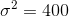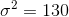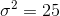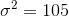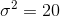Explanation:

Becausehas a Poisson distribution we know that: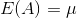and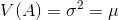.

Therefore, since we are given the mean of 25, we can find its variance to also be 25.